Problem

# Solutions For Applied Combinatorics Chapter 1.2 Problem 2E

#### Step-by-Step Solution

Solution 1

To list all nonisomorphic directed graphs with 3 vertices, do the following. First note that there are 6 possible edges. Now consider the cases where number of edges range from 0 to 6. For each case, draw all possible graphs, and check if any are isomorphic to any other. Keep only one graph from each set of isomorphic graphs.

Case 1: 0 edges. In this case, only one graph is possible. It is: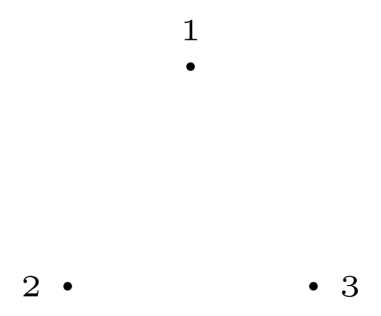Case 2: 1 edge. In this case, only one graph is possible, as there is only one edge. It is: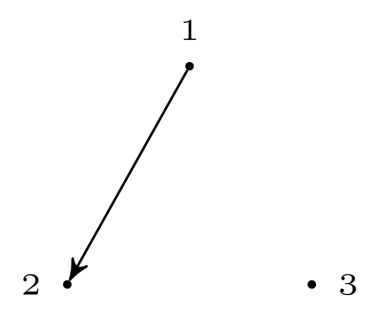Case 3: 2 edges. As there are only 3 vertices, the edges have to share an endpoint. Thus, they may share one or two endpoints. When they share two endpoints, only one case is possible.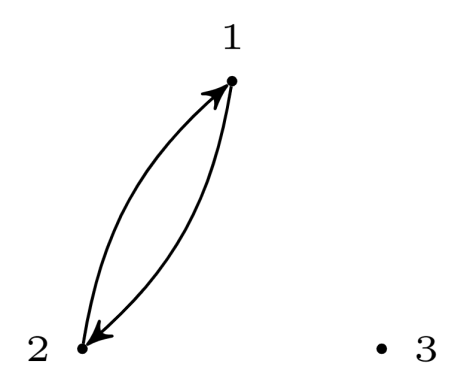When they share 1 endpoint, there are several cases to consider. The shared vertex might be the incoming vertex for both, outgoing vertex for both, or incoming for one and outgoing for the other. Hence the three possible graphs are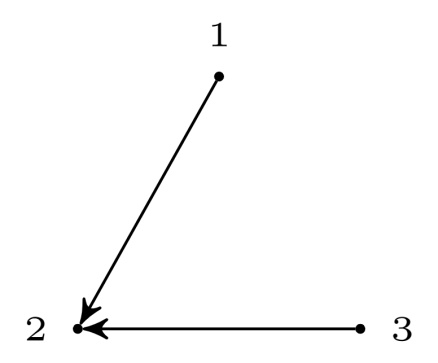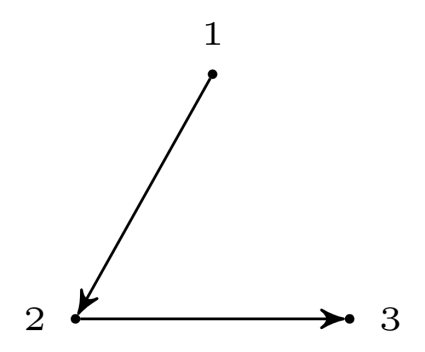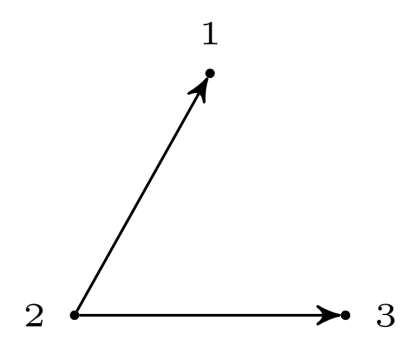Case 4: 3 edges. The three edges can either form a triangle, or two of the edges can share two endpoints.

When the three edges form a triangle, there are two possible cases. Either the edges can share the same orientation, or one edge can have opposite orientation to the other. The graphs are: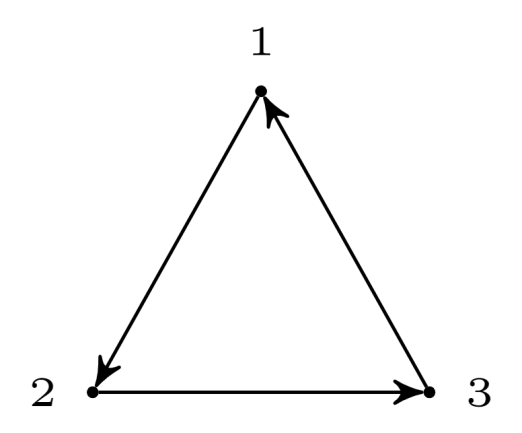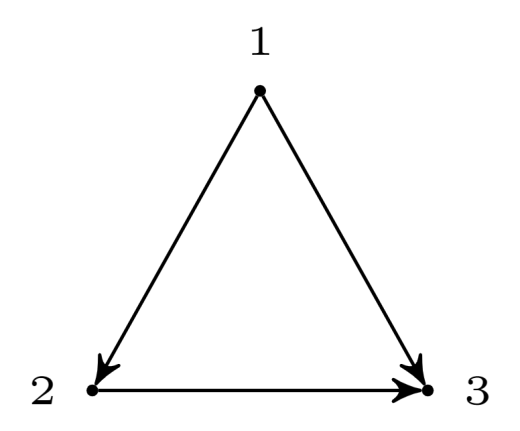When two edges share two endpoints, then the third edge has two possible orientations, towards the edges or away from them. The two graphs are: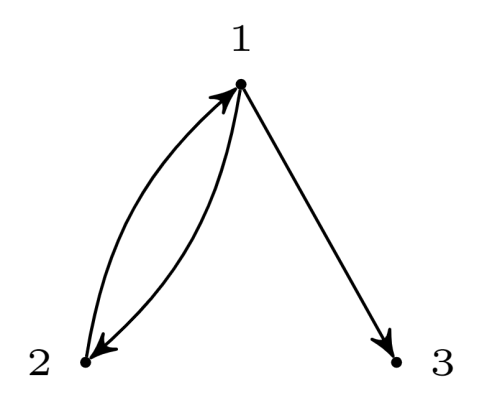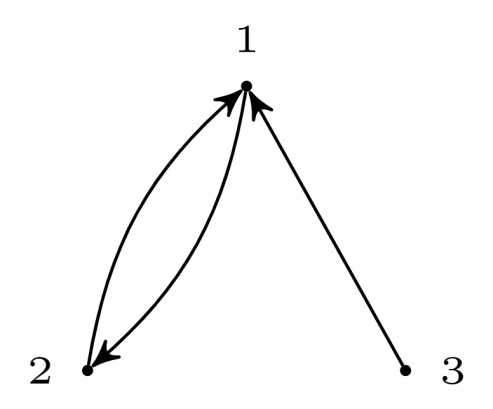Case 5: 4 edges. This is similar to Case 3, as the non-edges can be considered to be edges from Case 3, hence there are 4 graphs. The graphs are: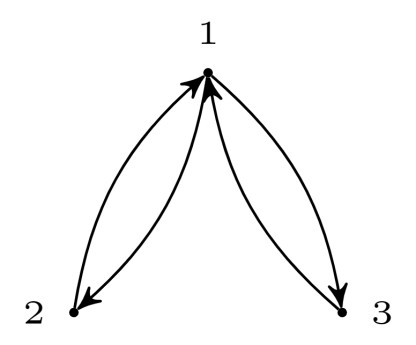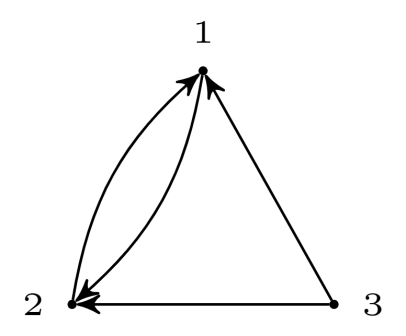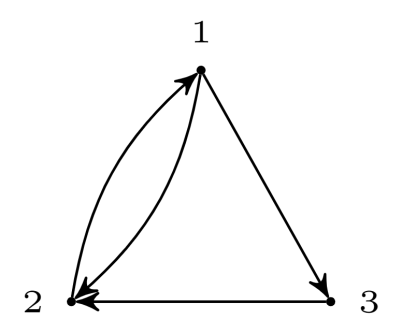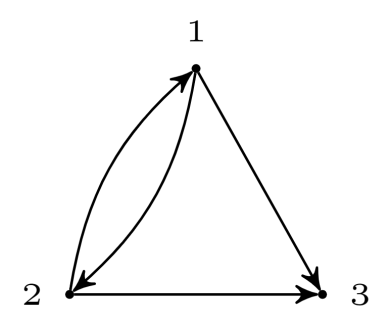Case 6: 5 edges. This is similar to Case 2, as non-edges can be considered as edges from Case 2. Hence there is only one graph which is: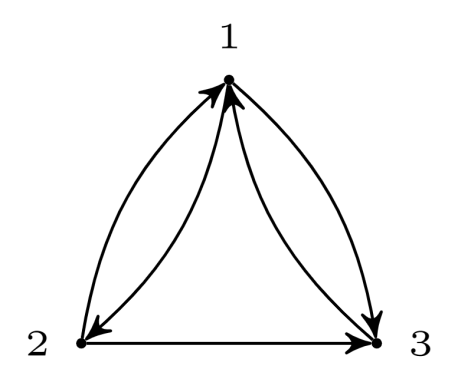Case 7: 6 edges. As all possible edges are present, there is only one possible case. It is: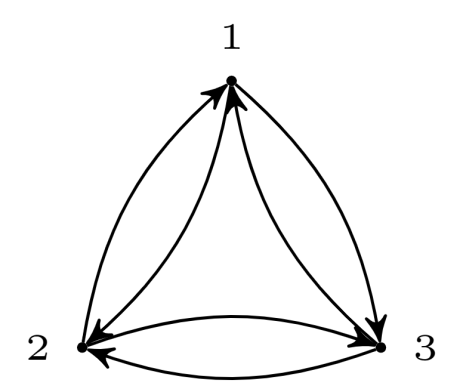These are all the possible cases.